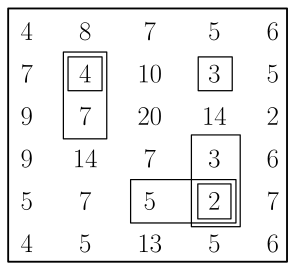# #534. 【IOI2019】矩形区域

19 世纪初，统治者下令在俯瞰美丽河景的高原上建造一座宫殿。这块高原被看做是一个由正方形单元格组成的 $n \times m$ 网格。网格的行从 $0$ 到 $n-1$ 编号，列从 $0$ 到 $m-1$ 编号。第 $i$ 行第 $j$ 列 $(0 \le i \le n-1,0 \le j \le m-1)$的单元格记为单元格 $(i,j)$ 。每个单元格 $(i,j)$ 有特定的海拔高度，记为 $a_{i,j}$。

### 样例一

#### input

6 5
4 8 7 5 6
7 4 10 3 5
9 7 20 14 2
9 14 7 3 6
5 7 5 2 7
4 5 13 5 6

#### output

6

#### explanation• $r1=r2=1,c1=c2=1$
• $r1=1,r2=2,c1=c2=1$
• $r1=r2=1,c1=c2=3$
• $r1=r2=1,c1=2,c2=3$
• $r1=r2=4,c1=c2=3$
• $r1=3,r2=4,c1=c2=1$

$a_{1,1}=4$ 严格小于 $a_{0,1}=8$，$a_{3,1}=14$，$a_{1,0}=7$和 $a_{1,2}=10$。 $a_{2,1}=7$ 严格小于 $a_{0,1}=8$，$a_{3,1}=14$，$a_{2,0}=9$和 $a_{2,2}=20$。

### 数据范围

$1$$n,m \le 308 2$$n,m \le 80$7
$3$$n,m \le 20012 4$$n,m \le 700$22
$5$$n \le 310 6$$a_{i,j} \le 1$13
$7$无特殊限制28